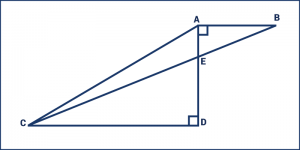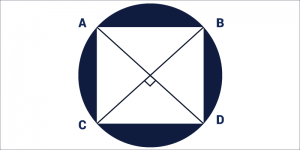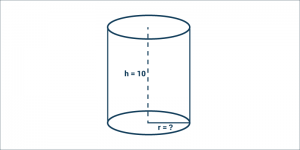# GMAT Geometry Sample Questions

Geometry questions in GMAT revolves around the following topics – Lines, Angles, Triangles, Special Right Triangles, Quadrilaterals, Circles, Polygons, Coordinate Geometry. It’s highly recommended that to start preparing for GMAT quant, clear your basics. To do so, grab the school books. You have learnt all of these during your schooling so just turn over the pages of maths books and clear your basics like – concepts and formulas. Here are few GMAT geometry sample questions for a better understanding on GMAT quant section –

Also Read: GMAT Quant: Geometry – Lines

## GMAT Geometry Questions

1. Find the value of x° in the following figure –Explanation – Since, line P and Q are parallel to each other, the transverse R will form same angle in both the line. Hence y° = 55°

And x° + y° = 180°

x° = 180° – 55°

x° = 125°

1. A circle with a radius of 7 has a square inscribed. Find the area of the shaded portion.As per traditional method you can subtract the area of the square from the area of the circle

but our motto is to save time on every quant questions.

So, let’s first find the area of the circle

which is A =

$\pi \; r^{2} = \pi \; 7^{2} = 49 \; \pi$

Use pythagorean theorem to find the length of the sides of the square.

$7^{2} + 7^{2} = a^{2}$

where a = base of the triangle = side of the square

$a^{2} = 49 + 49 = 98$ $a = \sqrt{98}$

Subtract the area of the square from the area of the circle to find the area of the shaded region.

Hence, the correct answer is 49π – 98

1. In the above figure AD = 8, AB = 6 and CD = 18. What is the area of triangle AEC?In the triangle AEC. let’s assume that AE is the base, and CD is the height.

Thus, CD = 9

To find the base we need to see that triangles AEB and CDE are similar. The ratio AB: CD, is therefore equal to the ratio AE: ED. The given information shows that the ratio is 6:18 or 1:3

Now dividing AD (8) in this ratio gives us AE as 1.

The area of AEC = $\frac{1}{2}\times base \times height$ $\frac{1}{2}\times 1 \times 18 = 9$

1. If square ABCD is inscribed inside the circle and DB and AC are both diameters of the circle, what is the circumference of the circle if the perimeter of the square is $4(200)^{\frac{1}{2}}$?As the Perimeter of the Square is  $4(200)^{\frac{1}{2}}$, then each of it’s side, a = $(200)^{\frac{1}{2}}$

We can derive the radius of a circle by using Pythagoras Theorem. In any of the triangles inside the square (i.e. Right Triangle), radius of the circle = r

Then, $r^{2} + r^{2} = a^{2}$ $\Rightarrow r^{2} + r^{2} = (200^{\frac{1}{2}})^{2}$ $\Rightarrow 2r^{2} = 200$ $\Rightarrow r^{2} = 100$ $\Rightarrow r = 10$

Therefore, Circumference = C  = 2πr

C = 2π10 = 20π

1. A water tank, which is right circular cylinder in shape, has height of 10 meters. If it is being filled at a rate of 4π gallons a minute until it is filled after 10 minutes, what is the radius of the base of the cylinder?When the water tank is full, the volume of water in the tank will equal the volume of the cylinder. Every minute, 4π gallons of water occupy the tank. When the tank is full, the volume of the cylinder will be $10(min) \times 4\pi \left ( \frac{gal}{min} \right ) = 40 \pi gal$; as it took 10 minutes to fill the tank and every minute another 4π gallons is poured into the tank.

Volume = $V = \pi r^{2}h$ $\Rightarrow 40 \pi = h \pi r^{2} = 10 \pi r^{2}$ $\Rightarrow 4 = r^{2}$ $\Rightarrow r = 2$

Hence, the radius of the base is 2 meters.

Read more GMAT Quant related articles.

BYJU’S will be glad to help you in your GMAT preparation journey. You can ask for any assistance related to GMAT and MBA from us by calling us at +918884544444. You can write to us at gmat@byjus.com.